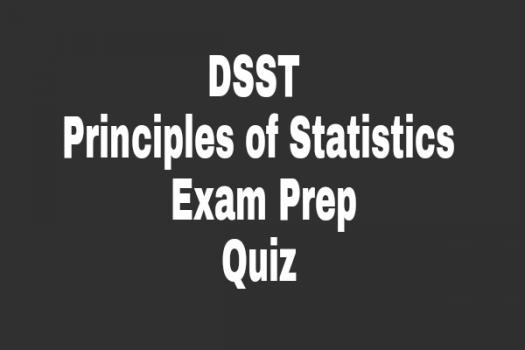# DSST Principles Of Statistics Exam Prep

10 Questions | Total Attempts: 108SettingsStatistics is a mathematical discipline that is concerned with the collection and interpretation of data. Modern statistical approaches involves the use of analytical software to effectively understand raw data and make a reasonable conclusion. This can be achieved by correct, data visualization. Evaluate your statistical reasoning by taking this quiz.

Related Topics
• 1.
What part of statistics deals with the analysis of random phenomena?
• A.

Standard deviation

• B.

Probability theory

• C.

Dispersion

• D.

Descriptive statistics

• 2.
What part of statistics focuses the variation within a distribution?
• A.

Central tendency

• B.

Observational study

• C.

Hypothesis

• D.

Dispersion

• 3.
Which of these is not related to statistics?
• A.

Linear algebra

• B.

Geometry

• C.

Differential equations

• D.

Stochastic analysis

• 4.
Which of these is not a numerical descriptor of a distribution?
• A.

Median

• B.

Mode

• C.

T-test

• D.

Mean

• 5.
Which of these is not a type of data?
• A.

Integer

• B.

Continuous

• C.

Categorical

• D.

Boolean

• 6.
Which of these is not an estimator?
• A.

Mode

• B.

Variance

• C.

Mean

• D.

Covariance

• 7.
Which of these random variables is not a pivot?
• A.

T value

• B.

Chi square statistic

• C.

F test

• D.

Z test

• 8.
When was the earliest work in statistics began?
• A.

1667

• B.

1663

• C.

1671

• D.

1563

• 9.
Which of these persons was not involved in development of probability theory?
• A.

Blaise Pascal

• B.

Pierre de Fermat

• C.

Gerolamo Cardano

• D.

Isaac Newton

• 10.
When was the method of least squares first discussed?
• A.

1908

• B.

1598

• C.

1784

• D.

1605